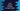# Java Program to check if a number is a magic number or not## Java program to check if a number is a magic number or not:

In this post, we will learn how to check if a number is magic number or not. This program will get one number as input from the user and print one message either it is a magic number or it is not a magic number.

I will show you different ways to solve this problem.

### What is a magic number:

A number is called a magic number if the sum of the digits of a number, if we find the sum repeatedly till we find a single digit is 1. For example, let’s check these numbers:

• 1234. The sum of its digits is 10. Since 10 is not one digit, we need to find the sum of the digits again which is 1 + 0 = 1, which is a one digit and equal to 1. So, it is a magic number.
• 1235. The sum of the digits is 11. Since 11 is not one digit, 1 + 1 is 2. So, it is not a magic number.

### Method 1: Java program to check if a number is a magic number or not:

In this program, we are taking a number as input from the user and finding the sum of its digits repeatedly until it become a single digit:

``````import java.util.Scanner;

class Main {
public static void main(String[] args) {
int no, sum = 0;
Scanner sc = new Scanner(System.in);

System.out.println("Enter a number: ");
no = sc.nextInt();

while (no > 0 || sum > 9) {
if (no == 0) {
no = sum;
sum = 0;
}
sum += no % 10;
no /= 10;
}

if (sum == 1) {
System.out.println("It is a Magic number");
} else {
System.out.println("It is not a Magic number");
}

}
}``````

In this program,

• no is an integer variable to hold the value of the user input number.
• sum is to hold the value of the sum of digits of the number.
• sc is a Scanner variable to read the user input.
• It asks the user to enter a number. It reads it and stores it in the no variable.
• The while loop will run till the value of no is positive or the value of sum is greater than 9, i.e. not one digit.
• If the value of no become 0, it assigns the calculated sum to no and reset the sum variable by assigning 0 to it.
• On each iteration, it gets the last digit of no by using % 10 and adds that value to sum.
• It also removes the last digit from no on each iteration.
• Once the loop ends, it checks the value of sum. If it is 1, it is a Magic number, else it is not a Magic number.

If you run this program, it will print output as like below:

``````Enter a number:
1235
It is not a Magic number

Enter a number:
1234
It is a Magic number``````

### Method 2: Check if a number is Magic number or not by using a separate method:

We can also use a separate method to check if a number is Magic number or not.

``````import java.util.Scanner;

class Main {
public static boolean isMagicNumber(int no) {
int sum = 0;
while (no > 0 || sum > 9) {
if (no == 0) {
no = sum;
sum = 0;
}
sum += no % 10;
no /= 10;
}

return sum == 1;
}

public static void main(String[] args) {
int no;
Scanner sc = new Scanner(System.in);

System.out.println("Enter a number: ");
no = sc.nextInt();

if (isMagicNumber(no)) {
System.out.println("It is a Magic number");
} else {
System.out.println("It is not a Magic number");
}
}
}``````

Here,

• We created a new method called isMagicNumber.
• This method takes one number as the parameter and returns one boolean value.
• It finds the sum of the digits of the number and returns true if it is 1, else it returns false.

It will give a similar result.